Theory and Modern Applications

# Some Volterra-Fredholm type nonlinear discrete inequalities involving four iterated infinite sums

## Abstract

Some new generalized Volterra-Fredholm type nonlinear discrete inequalities involving four iterated infinite sums are established in this paper. To illustrate the validity of the established inequalities, we present some applications for them, in which new explicit bounds for the solutions of certain infinite sum-difference equations are deduced.

MSC:26D15.

## 1 Introduction

In recent years, many researchers have focused on various generalizations of the known Gronwall-Bellman inequality [1, 2], which provide explicit bounds for unknown solutions of certain difference equations, and a lot of such generalized inequalities have been established in the literature  including the known Ou-Iang inequality . In , Ma generalized the discrete version of Ou-Iang’s inequality in two variables to a Volterra-Fredholm form for the first time, which has proved to be very useful in the study of qualitative as well as quantitative properties of the solutions of certain Volterra-Fredholm type difference equations. But since then, few results on Volterra-Fredholm type discrete inequalities have been established. Recent results in this direction include the works of Zheng , Ma , Zheng and Feng  to our best knowledge. We notice that the Volterra-Fredholm type discrete inequalities in  are constructed by an explicit function ${u}^{p}$ in the left-hand side (see [, Theorems 2.5, 2.6], [, Theorems 2.1, 2.5, 2.6, 2.7], [, Theorems 5, 8, 10, 11]).

Motivated by the works in , in this paper, we establish some new generalized Volterra-Fredholm type discrete inequalities involving four iterated infinite sums with the right-hand side denoted by an arbitrary function $\varphi \left(u\right)$, which are of more general forms. To illustrate the usefulness of the established results, we also present some applications for them and study the boundedness of the solutions of certain Volterra-Fredholm type infinite sum-difference equations.

Throughout this paper, denotes the set of real numbers and ${\mathbb{R}}_{+}=\left[0,\mathrm{\infty }\right)$, and denotes the set of integers, while ${\mathbb{N}}_{0}$ denotes the set of nonnegative integers. In the next of this paper, let $\mathrm{\Omega }:=\left(\left[{m}_{0},\mathrm{\infty }\right]×\left[{n}_{0},\mathrm{\infty }\right]\right)\cap {\mathbb{Z}}^{2}$, where ${m}_{0},{n}_{0}\in \mathbb{Z}$, and let ${l}_{1},{l}_{2}\in \mathbb{Z}$ be two constants. If U is a lattice, then we denote the set of all -valued functions on U by $\mathrm{\wp }\left(U\right)$ and denote the set of all ${\mathbb{R}}_{+}$-valued functions on U by ${\mathrm{\wp }}_{+}\left(U\right)$. Finally, for a function $f\in {\mathrm{\wp }}_{+}\left(U\right)$, we have ${\sum }_{s={m}_{0}}^{{m}_{1}}f=0$ provided ${m}_{0}>{m}_{1}$.

## 2 Main results

Lemma 2.1 [, Lemma 2.1]

Suppose $u,a,b\in {\mathrm{\wp }}_{+}\left(\mathrm{\Omega }\right)$. If $a\left(m,n\right)$ is nonincreasing in the first variable, then for $\left(m,n\right)\in \mathrm{\Omega }$,

$u\left(m,n\right)\le a\left(m,n\right)+\sum _{s=m+1}^{\mathrm{\infty }}b\left(s,n\right)u\left(s,n\right)$

implies

$u\left(m,n\right)\le a\left(m,n\right)\sum _{s=m+1}^{\mathrm{\infty }}\left[1+b\left(s,n\right)\right].$
(1)

Lemma 2.2 Suppose $u,a,H\in {\mathrm{\wp }}_{+}\left(\mathrm{\Omega }\right)$, $b\in {\mathrm{\wp }}_{+}\left({\mathrm{\Omega }}^{2}\right)$, and H, a are nonincreasing in every variable with $H\left(m,n\right)>0$, while b is nonincreasing in the third variable. $\phi ,\varphi \in C\left({\mathbb{R}}_{+},{\mathbb{R}}_{+}\right)$ are strictly increasing with $\phi \left(r\right)>0$, $\varphi \left(r\right)>0$ for $r>0$. If for $\left(m,n\right)\in \mathrm{\Omega }$, $u\left(m,n\right)$ satisfies the following inequality:

$u\left(m,n\right)\le H\left(m,n\right)+\sum _{s=m+1}^{\mathrm{\infty }}\sum _{t=n+1}^{\mathrm{\infty }}b\left(s,t,m,n\right)\phi \left({\varphi }^{-1}\left(u\left(s,t\right)+a\left(s,t\right)\right)\right),$
(2)

then we have

$u\left(m,n\right)\le {G}^{-1}\left[G\left(H\left(m,n\right)\right)+\sum _{s=m+1}^{\mathrm{\infty }}\sum _{t=n+1}^{\mathrm{\infty }}b\left(s,t,m,n\right)\right],$
(3)

where

$G\left(z\right)={\int }_{{z}_{0}}^{z}\frac{1}{\phi \left[{\varphi }^{-1}\left(z+a\left(m,n\right)\right)\right]}\phantom{\rule{0.2em}{0ex}}dz,\phantom{\rule{1em}{0ex}}z\ge {z}_{0}>0.$
(4)

Proof Fix $\left({m}_{1},{n}_{1}\right)\in \mathrm{\Omega }$, and let $\left(m,n\right)\in \left(\left[{m}_{1},\mathrm{\infty }\right]×\left[{n}_{1},\mathrm{\infty }\right]\right)\cap \mathrm{\Omega }$. Then we have

$u\left(m,n\right)\le H\left({m}_{1},{n}_{1}\right)+\sum _{s=m+1}^{\mathrm{\infty }}\sum _{t=n+1}^{\mathrm{\infty }}b\left(s,t,m,n\right)\phi \left[{\varphi }^{-1}\left(u\left(s,t\right)+a\left(s,t\right)\right)\right].$
(5)

Let the right-hand side of (5) be $v\left(m,n\right)$. Then

$u\left(m,n\right)\le v\left(m,n\right),\phantom{\rule{1em}{0ex}}\left(m,n\right)\in \left(\left[{m}_{1},\mathrm{\infty }\right]×\left[{n}_{1},\mathrm{\infty }\right]\right)\cap \mathrm{\Omega },$
(6)

and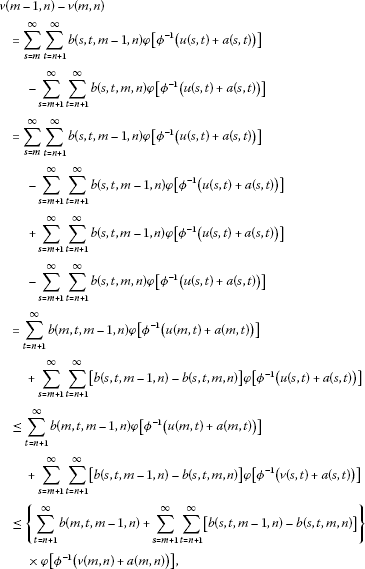that is,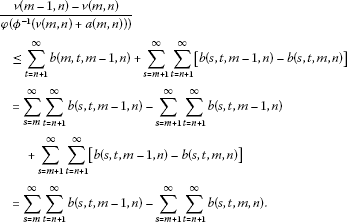(7)

On the other hand, according to the mean-value theorem for integrals, there exists ξ such that $v\left(m,n\right)\le \xi \le v\left(m-1,n\right)$, and

$\begin{array}{rcl}{\int }_{v\left(m,n\right)}^{v\left(m-1,n\right)}\frac{1}{\phi \left({\varphi }^{-1}\left(z+a\left(m,n\right)\right)\right)}\phantom{\rule{0.2em}{0ex}}dz& =& \frac{v\left(m-1,n\right)-v\left(m,n\right)}{\phi \left({\varphi }^{-1}\left(\xi +a\left(m,n\right)\right)\right)}\\ \le & \frac{v\left(m-1,n\right)-v\left(m,n\right)}{\phi \left({\varphi }^{-1}\left(v\left(m,n\right)+a\left(m,n\right)\right)\right)}.\end{array}$
(8)

So, combining (7) and (8), we have

$\begin{array}{rcl}{\int }_{v\left(m,n\right)}^{v\left(m-1,n\right)}\frac{1}{\phi \left({\varphi }^{-1}\left(z+a\left(m,n\right)\right)\right)}\phantom{\rule{0.2em}{0ex}}dz& =& G\left(v\left(m-1,n\right)\right)-G\left(v\left(m,n\right)\right)\\ \le & \sum _{s=m}^{\mathrm{\infty }}\sum _{t=n+1}^{\mathrm{\infty }}b\left(s,t,m-1,n\right)-\sum _{s=m+1}^{\mathrm{\infty }}\sum _{t=n+1}^{\mathrm{\infty }}b\left(s,t,m,n\right),\end{array}$
(9)

where G is defined in (4). Set $m=\eta$ in (9); a summation with respect to η from $m+1$ to ∞ yields

$G\left(v\left(m,n\right)\right)-G\left(v\left(\mathrm{\infty },n\right)\right)\le \sum _{s=m+1}^{\mathrm{\infty }}\sum _{t=n+1}^{\mathrm{\infty }}b\left(s,t,m,n\right)-0=\sum _{s=m+1}^{\mathrm{\infty }}\sum _{t=n+1}^{\mathrm{\infty }}b\left(s,t,m,n\right).$

Noticing $v\left(\mathrm{\infty },n\right)=H\left({m}_{1},{n}_{1}\right)$ and G is increasing, it follows that

$v\left(m,n\right)\le {G}^{-1}\left[G\left(H\left({m}_{1},{n}_{1}\right)\right)+\sum _{s=m+1}^{\mathrm{\infty }}\sum _{t=n+1}^{\mathrm{\infty }}b\left(s,t,m,n\right)\right].$
(10)

Combining (6) and (10), we obtain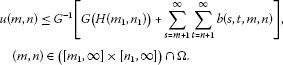(11)

Setting $m={m}_{1}$, $n={n}_{1}$ in (11) yields

$u\left({m}_{1},{n}_{1}\right)\le {G}^{-1}\left[G\left(a\left({m}_{1},{n}_{1}\right)\right)+\sum _{s={m}_{1}+1}^{\mathrm{\infty }}\sum _{t={n}_{1}+1}^{\mathrm{\infty }}b\left(s,t,{m}_{1},{n}_{1}\right)\right].$
(12)

Since $\left({m}_{1},{n}_{1}\right)$ is selected from Ω arbitrarily, then substituting $\left({m}_{1},{n}_{1}\right)$ with $\left(m,n\right)$ in (12), we get the desired inequality (3). □

Theorem 2.3 Suppose $u\in {\mathrm{\wp }}_{+}\left(\mathrm{\Omega }\right)$, ${b}_{i},{c}_{i}\in {\mathrm{\wp }}_{+}\left({\mathrm{\Omega }}^{2}\right)$, $i=1,2,\dots ,{l}_{1}$, ${d}_{i},{e}_{i}\in {\mathrm{\wp }}_{+}\left({\mathrm{\Omega }}^{2}\right)$, $i=1,2,\dots ,{l}_{2}$ with ${b}_{i}$, ${c}_{i}$, ${d}_{i}$, ${e}_{i}$ nonincreasing in the last two variables, and there is at least one function among ${d}_{i}$, ${e}_{i}$, $i=1,2,\dots ,{l}_{2}$ not equivalent to zero, a, φ, ϕ are defined as in Lemma  2.2. If for $\left(m,n\right)\in \mathrm{\Omega }$, $u\left(m,n\right)$ satisfies

$\begin{array}{rcl}\varphi \left(u\left(m,n\right)\right)& \le & a\left(m,n\right)+\sum _{i=1}^{{l}_{1}}\sum _{s=m+1}^{\mathrm{\infty }}\sum _{t=n+1}^{\mathrm{\infty }}\left[{b}_{i}\left(s,t,m,n\right)\phi \left(u\left(s,t\right)\right)\\ +\sum _{\xi =s}^{\mathrm{\infty }}\sum _{\eta =t}^{\mathrm{\infty }}{c}_{i}\left(\xi ,\eta ,m,n\right)\phi \left(u\left(\xi ,\eta \right)\right)\right]\\ +\sum _{i=1}^{{l}_{2}}\sum _{s=M+1}^{\mathrm{\infty }}\sum _{t=N+1}^{\mathrm{\infty }}\left[{d}_{i}\left(s,t,m,n\right)\varphi \left(u\left(s,t\right)\right)\\ +\sum _{\xi =s}^{\mathrm{\infty }}\sum _{\eta =t}^{\mathrm{\infty }}{e}_{i}\left(\xi ,\eta ,m,n\right)\varphi \left(u\left(\xi ,\eta \right)\right)\right],\end{array}$
(13)

then

$\begin{array}{rcl}u\left(m,n\right)& \le & {\varphi }^{-1}\left\{a\left(m,n\right)+{G}^{-1}\left\{G\left({T}^{-1}\left[\sum _{s={m}_{0}}^{M-1}\sum _{t={n}_{0}}^{N-1}B\left(s,t,M,N\right)\right]\right)\\ +\sum _{s=m+1}^{\mathrm{\infty }}\sum _{t=n+1}^{\mathrm{\infty }}B\left(s,t,m,n\right)\right\}\right\}\end{array}$
(14)

provided that T is increasing, where G is defined in (4), and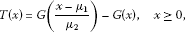(15)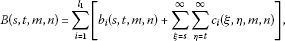(16)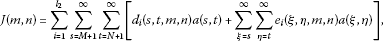(17)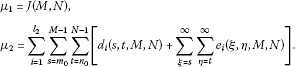(18)

Proof Denote

$\begin{array}{rcl}v\left(m,n\right)& =& \sum _{i=1}^{{l}_{1}}\sum _{s=m+1}^{\mathrm{\infty }}\sum _{t=n+1}^{\mathrm{\infty }}\left[{b}_{i}\left(s,t,m,n\right)\phi \left(u\left(s,t\right)\right)+\sum _{\xi =s}^{\mathrm{\infty }}\sum _{\eta =t}^{\mathrm{\infty }}{c}_{i}\left(\xi ,\eta ,m,n\right)\phi \left(u\left(\xi ,\eta \right)\right)\right]\\ +\sum _{i=1}^{{l}_{2}}\sum _{s=M+1}^{\mathrm{\infty }}\sum _{t=N+1}^{\mathrm{\infty }}\left[{d}_{i}\left(s,t,m,n\right)\varphi \left(u\left(s,t\right)\right)+\sum _{\xi =s}^{\mathrm{\infty }}\sum _{\eta =t}^{\mathrm{\infty }}{e}_{i}\left(\xi ,\eta ,m,n\right)\varphi \left(u\left(\xi ,\eta \right)\right)\right].\end{array}$

Then we have

$u\left(m,n\right)\le {\varphi }^{-1}\left(a\left(m,n\right)+v\left(m,n\right)\right).$
(19)

So,

$\begin{array}{rcl}v\left(m,n\right)& \le & \sum _{i=1}^{{l}_{1}}\sum _{s=m+1}^{\mathrm{\infty }}\sum _{t=n+1}^{\mathrm{\infty }}\left\{{b}_{i}\left(s,t,m,n\right)\phi \left[{\varphi }^{-1}\left(a\left(s,t\right)+v\left(s,t\right)\right)\right]\\ +\sum _{\xi =s}^{\mathrm{\infty }}\sum _{\eta =t}^{\mathrm{\infty }}{c}_{i}\left(\xi ,\eta ,m,n\right)\phi \left[{\varphi }^{-1}\left(a\left(\xi ,\eta \right)+v\left(\xi ,\eta \right)\right)\right]\right\}\\ +\sum _{i=1}^{{l}_{2}}\sum _{s=M+1}^{\mathrm{\infty }}\sum _{t=N+1}^{\mathrm{\infty }}\left[{d}_{i}\left(s,t,m,n\right)\left(a\left(s,t\right)+v\left(s,t\right)\right)\\ +\sum _{\xi =s}^{\mathrm{\infty }}\sum _{\eta =t}^{\mathrm{\infty }}{e}_{i}\left(\xi ,\eta ,m,n\right)\left(a\left(\xi ,\eta \right)+v\left(\xi ,\eta \right)\right)\right]\\ =& H\left(m,n\right)+\sum _{i=1}^{{l}_{1}}\sum _{s=m+1}^{\mathrm{\infty }}\sum _{t=n+1}^{\mathrm{\infty }}\left\{{b}_{i}\left(s,t,m,n\right)\phi \left[{\varphi }^{-1}\left(a\left(s,t\right)+v\left(s,t\right)\right)\right]\\ +\sum _{\xi =s}^{\mathrm{\infty }}\sum _{\eta =t}^{\mathrm{\infty }}{c}_{i}\left(\xi ,\eta ,m,n\right)\phi \left[{\varphi }^{-1}\left(a\left(\xi ,\eta \right)+v\left(\xi ,\eta \right)\right)\right]\right\},\end{array}$
(20)

where $H\left(m,n\right)=J\left(m,n\right)+{\sum }_{i=1}^{{l}_{2}}{\sum }_{s=M+1}^{\mathrm{\infty }}{\sum }_{t=N+1}^{\mathrm{\infty }}\left[{d}_{i}\left(s,t,m,n\right)v\left(s,t\right)+{\sum }_{\xi =s}^{\mathrm{\infty }}{\sum }_{\eta =t}^{\mathrm{\infty }}{e}_{i}\left(\xi ,\eta ,m,n\right)v\left(\xi ,\eta \right)\right]$, and $J\left(m,n\right)$ is defined in (17). Then using $H\left(m,n\right)$ is nonincreasing in every variable, we obtain

$\begin{array}{rcl}v\left(m,n\right)& \le & H\left(M,N\right)+\sum _{i=1}^{{l}_{1}}\sum _{s=m+1}^{\mathrm{\infty }}\sum _{t=n+1}^{\mathrm{\infty }}\left\{{b}_{i}\left(s,t,m,n\right)\phi \left[{\varphi }^{-1}\left(a\left(s,t\right)+v\left(s,t\right)\right)\right]\right\}\\ +\sum _{\xi =s}^{\mathrm{\infty }}\sum _{\eta =t}^{\mathrm{\infty }}{c}_{i}\left(\xi ,\eta ,m,n\right)\phi \left[{\varphi }^{-1}\left(a\left(\xi ,\eta \right)+v\left(\xi ,\eta \right)\right)\right]\\ \le & H\left(M,N\right)+\sum _{i=1}^{{l}_{1}}\sum _{s=m+1}^{\mathrm{\infty }}\sum _{t=n+1}^{\mathrm{\infty }}\left[{b}_{i}\left(s,t,m,n\right)+\sum _{\xi =s}^{\mathrm{\infty }}\sum _{\eta =t}^{\mathrm{\infty }}{c}_{i}\left(\xi ,\eta ,m,n\right)\right]\\ ×\phi \left[{\varphi }^{-1}\left(a\left(s,t\right)+v\left(s,t\right)\right)\right]\\ =& H\left(M,N\right)+\sum _{s=m+1}^{\mathrm{\infty }}\sum _{t=n+1}^{\mathrm{\infty }}B\left(s,t,m,n\right)\phi \left[{\varphi }^{-1}\left(a\left(s,t\right)+v\left(s,t\right)\right)\right],\end{array}$
(21)

where $B\left(s,t,m,n\right)$ is defined in (16).

Since there is at least one function among ${d}_{i}$, ${e}_{i}$, $i=1,2,\dots ,{l}_{2}$ not equivalent to zero, then $H\left(M,N\right)>0$. On the other hand, as ${b}_{i}\left(s,t,m,n\right)$, ${c}_{i}\left(s,t,m,n\right)$ are both nonincreasing in the last two variables, then $B\left(s,t,m,n\right)$ is also nonincreasing in the last two variables, and by a suitable application of Lemma 2.2, we obtain

$v\left(m,n\right)\le {G}^{-1}\left[G\left(H\left(M,N\right)\right)+\sum _{s=m+1}^{\mathrm{\infty }}\sum _{t=n+1}^{\mathrm{\infty }}B\left(s,t,m,n\right)\right].$
(22)

Furthermore, by the definitions of $H\left(m,n\right)$, ${\mu }_{1}$, ${\mu }_{2}$ and (22), we have

$\begin{array}{rcl}H\left(M,N\right)& =& J\left(M,N\right)+\sum _{i=1}^{{l}_{2}}\sum _{s=M+1}^{\mathrm{\infty }}\sum _{t=N+1}^{\mathrm{\infty }}\left\{{d}_{i}\left(s,t,M,N\right)v\left(s,t\right)\\ +\sum _{\xi =s}^{\mathrm{\infty }}\sum _{\eta =t}^{\mathrm{\infty }}{e}_{i}\left(\xi ,\eta ,M,N\right)v\left(\xi ,\eta \right)\right\}\\ \le & J\left(M,N\right)+v\left(M,N\right)\sum _{i=1}^{{l}_{2}}\sum _{s=M+1}^{\mathrm{\infty }}\sum _{t=N+1}^{\mathrm{\infty }}\left\{{d}_{i}\left(s,t,M,N\right)+\sum _{\xi =s}^{\mathrm{\infty }}\sum _{\eta =t}^{\mathrm{\infty }}{e}_{i}\left(\xi ,\eta ,M,N\right)\right\}\\ =& {\mu }_{1}+{\mu }_{2}v\left(M,N\right)\\ \le & {\mu }_{1}+{\mu }_{2}{G}^{-1}\left[G\left(H\left(M,N\right)\right)+\sum _{s=M+1}^{\mathrm{\infty }}\sum _{t=N+1}^{\mathrm{\infty }}B\left(s,t,M,N\right)\right],\end{array}$

and

$G\left(\frac{H\left(M,N\right)-{\mu }_{1}}{{\mu }_{2}}\right)\le G\left(H\left(M,N\right)\right)+\sum _{s=M+1}^{\mathrm{\infty }}\sum _{t=N+1}^{\mathrm{\infty }}B\left(s,t,M,N\right),$

which is rewritten as

$T\left(H\left(M,N\right)\right)\le \sum _{s=M+1}^{\mathrm{\infty }}\sum _{t=N+1}^{\mathrm{\infty }}B\left(s,t,M,N\right),$

where T is defined in (15). By T is increasing, we have

$H\left(M,N\right)\le {T}^{-1}\left[\sum _{s=M+1}^{\mathrm{\infty }}\sum _{t=N+1}^{\mathrm{\infty }}B\left(s,t,M,N\right)\right].$
(23)

Combining (19), (22) and (23), we get the desired result. □

Corollary 2.4 Suppose ${g}_{1i},{g}_{2i},{b}_{1i},{c}_{1i}\in {\mathrm{\wp }}_{+}\left(\mathrm{\Omega }\right)$, $i=1,2,\dots ,{l}_{1}$ with ${g}_{1i}$, ${g}_{2i}$ nonincreasing in every variable, ${d}_{1i},{e}_{1i}\in {\mathrm{\wp }}_{+}\left(\mathrm{\Omega }\right)$, $i=1,2,\dots ,{l}_{2}$, u, a, φ, ϕ are defined as in Theorem  2.3. If for $\left(m,n\right)\in \mathrm{\Omega }$, $u\left(m,n\right)$ satisfies

$\begin{array}{rcl}\varphi \left(u\left(m,n\right)\right)& \le & a\left(m,n\right)+\sum _{i=1}^{{l}_{1}}{g}_{1i}\left(m,n\right)\sum _{s=m+1}^{\mathrm{\infty }}\sum _{t=n+1}^{\mathrm{\infty }}\left[{b}_{1i}\left(s,t\right)\phi \left(u\left(s,t\right)\right)\\ +\sum _{\xi =s}^{\mathrm{\infty }}\sum _{\eta =t}^{\mathrm{\infty }}{c}_{1i}\left(\xi ,\eta \right)\phi \left(u\left(\xi ,\eta \right)\right)\right]\\ +\sum _{i=1}^{{l}_{2}}{g}_{2i}\left(m,n\right)\sum _{s=M+1}^{\mathrm{\infty }}\sum _{t=N+1}^{\mathrm{\infty }}\left[{d}_{1i}\left(s,t\right)\varphi \left(u\left(s,t\right)\right)+\sum _{\xi =s}^{\mathrm{\infty }}\sum _{\eta =t}^{\mathrm{\infty }}{e}_{1i}\left(\xi ,\eta \right)\varphi \left(u\left(\xi ,\eta \right)\right)\right],\end{array}$

then

$\begin{array}{rcl}u\left(m,n\right)& \le & {\varphi }^{-1}\left\{a\left(m,n\right)+{G}^{-1}\left\{G\left({T}^{-1}\left[\sum _{s=M+1}^{\mathrm{\infty }}\sum _{t=N+1}^{\mathrm{\infty }}B\left(s,t,M,N\right)\right]\right)\\ +\sum _{s=m+1}^{\mathrm{\infty }}\sum _{t=n+1}^{\mathrm{\infty }}B\left(s,t,m,n\right)\right\}\right\}\end{array}$

provided that T is increasing, where G, T are defined in Theorem  2.3, and

$\begin{array}{c}B\left(s,t,m,n\right)=\sum _{i=1}^{{l}_{1}}{g}_{1i}\left(m,n\right)\left[{b}_{i}\left(s,t\right)+\sum _{\xi =s}^{\mathrm{\infty }}\sum _{\eta =t}^{\mathrm{\infty }}{c}_{i}\left(\xi ,\eta \right)\right],\hfill \\ J\left(m,n\right)=\sum _{i=1}^{{l}_{2}}{g}_{2i}\left(m,n\right)\sum _{s=M+1}^{\mathrm{\infty }}\sum _{t=N+1}^{\mathrm{\infty }}\left[{d}_{i}\left(s,t\right)a\left(s,t\right)+\sum _{\xi =s}^{\mathrm{\infty }}\sum _{\eta =t}^{\mathrm{\infty }}{e}_{i}\left(\xi ,\eta \right)a\left(\xi ,\eta \right)\right],\hfill \\ {\mu }_{1}=J\left(M,N\right),\phantom{\rule{2em}{0ex}}{\mu }_{2}=\sum _{i=1}^{{l}_{2}}{g}_{2i}\left(m,n\right)\sum _{s=M+1}^{\mathrm{\infty }}\sum _{t=N+1}^{\mathrm{\infty }}\left[{d}_{i}\left(s,t\right)+\sum _{\xi =s}^{\mathrm{\infty }}\sum _{\eta =t}^{\mathrm{\infty }}{e}_{i}\left(\xi ,\eta \right)\right].\hfill \end{array}$

The proof for Corollary 2.4 can be completed by setting ${b}_{i}\left(s,t,m,n\right)={g}_{1i}\left(m,n\right){b}_{1i}\left(s,t\right)$, ${c}_{i}\left(s,t,m,n\right)={g}_{1i}\left(m,n\right){c}_{1i}\left(s,t\right)$, ${d}_{i}\left(s,t,m,n\right)={g}_{2i}\left(m,n\right){d}_{1i}\left(s,t\right)$, ${e}_{i}\left(s,t,m,n\right)={g}_{2i}\left(m,n\right){e}_{1i}\left(s,t\right)$ in Theorem 2.3.

Theorem 2.5 Suppose $w\in {\mathrm{\wp }}_{+}\left(\mathrm{\Omega }\right)$, u, a, ${b}_{i}$, ${c}_{i}$, ${d}_{i}$, ${e}_{i}$, φ, ϕ are defined as in Theorem  2.3. Furthermore, assume $\phi \circ {\varphi }^{-1}$ is submultiplicative, that is, $\phi \left({\varphi }^{-1}\left(\alpha \beta \right)\right)\le \phi \left({\varphi }^{-1}\left(\alpha \right)\right)\phi \left({\varphi }^{-1}\left(\beta \right)\right)$ $\mathrm{\forall }\alpha ,\beta \in {\mathbb{R}}_{+}$. If for $\left(m,n\right)\in \mathrm{\Omega }$, $u\left(m,n\right)$ satisfies

$\begin{array}{rcl}\varphi \left(u\left(m,n\right)\right)& \le & a\left(m,n\right)+\sum _{s=m+1}^{\mathrm{\infty }}w\left(s,n\right)\varphi \left(u\left(s,n\right)\right)\\ +\sum _{i=1}^{{l}_{1}}\sum _{s=m+1}^{\mathrm{\infty }}\sum _{t=n+1}^{\mathrm{\infty }}\left[{b}_{i}\left(s,t,m,n\right)\phi \left(u\left(s,t\right)\right)+\sum _{\xi =s}^{\mathrm{\infty }}\sum _{\eta =t}^{\mathrm{\infty }}{c}_{i}\left(\xi ,\eta ,m,n\right)\phi \left(u\left(\xi ,\eta \right)\right)\right]\\ +\sum _{i=1}^{{l}_{2}}\sum _{s=M+1}^{\mathrm{\infty }}\sum _{t=N+1}^{\mathrm{\infty }}\left[{d}_{i}\left(s,t,m,n\right)\varphi \left(u\left(s,t\right)\right)\\ +\sum _{\xi =s}^{\mathrm{\infty }}\sum _{\eta =t}^{\mathrm{\infty }}{e}_{i}\left(\xi ,\eta ,m,n\right)\varphi \left(u\left(\xi ,\eta \right)\right)\right],\end{array}$
(24)

then

$\begin{array}{rcl}u\left(m,n\right)& \le & {\varphi }^{-1}\left\{\left\{a\left(m,n\right)+{G}^{-1}\left\{G\left({T}^{-1}\left[\sum _{s=M+1}^{\mathrm{\infty }}\sum _{t=N+1}^{\mathrm{\infty }}\overline{B}\left(s,t,M,N\right)\right]\right)\\ +\sum _{i=1}^{{l}_{1}}\sum _{s=m+1}^{\mathrm{\infty }}\sum _{t=n+1}^{\mathrm{\infty }}\overline{B}\left(s,t,m,n\right)\right\}\right\}\overline{w}\left(m,n\right)\right\}\end{array}$
(25)

provided that T is increasing, where G is defined in (4), and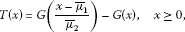(26)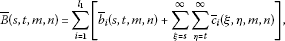(27)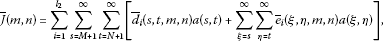(28)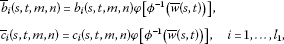(29)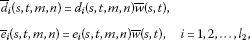(30)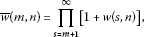(31)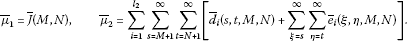(32)

Proof Denote

$\begin{array}{rcl}z\left(m,n\right)& =& a\left(m,n\right)+\sum _{i=1}^{{l}_{1}}\sum _{s=m+1}^{\mathrm{\infty }}\sum _{t=n+1}^{\mathrm{\infty }}\left[{b}_{i}\left(s,t,m,n\right)\phi \left(u\left(s,t\right)\right)\\ +\sum _{\xi =s}^{\mathrm{\infty }}\sum _{\eta =t}^{\mathrm{\infty }}{c}_{i}\left(\xi ,\eta ,m,n\right)\phi \left(u\left(\xi ,\eta \right)\right)\right]\\ +\sum _{i=1}^{{l}_{2}}\sum _{s=M+1}^{\mathrm{\infty }}\sum _{t=N+1}^{\mathrm{\infty }}\left[{d}_{i}\left(s,t,m,n\right)\varphi \left(u\left(s,t\right)\right)+\sum _{\xi =s}^{\mathrm{\infty }}\sum _{\eta =t}^{\mathrm{\infty }}{e}_{i}\left(\xi ,\eta ,m,n\right)\varphi \left(u\left(\xi ,\eta \right)\right)\right].\end{array}$

Then we have

$\varphi \left(u\left(m,n\right)\right)\le z\left(m,n\right)+\sum _{s=m+1}^{\mathrm{\infty }}w\left(s,n\right)\varphi \left(u\left(s,n\right)\right).$
(33)

Obviously, $z\left(m,n\right)$ is nonincreasing in the first variable. So, by Lemma 2.1, we obtain

$\varphi \left(u\left(m,n\right)\right)\le z\left(m,n\right)\prod _{s=m+1}^{\mathrm{\infty }}\left[1+w\left(s,n\right)\right]=z\left(m,n\right)\overline{w}\left(m,n\right),$

where $\overline{w}\left(m,n\right)$ is defined in (31). Define

$\begin{array}{rcl}v\left(m,n\right)& =& \sum _{i=1}^{{l}_{1}}\sum _{s=m+1}^{\mathrm{\infty }}\sum _{t=n+1}^{\mathrm{\infty }}\left[{b}_{i}\left(s,t,m,n\right)\phi \left(u\left(s,t\right)\right)+\sum _{\xi =s}^{\mathrm{\infty }}\sum _{\eta =t}^{\mathrm{\infty }}{c}_{i}\left(\xi ,\eta ,m,n\right)\phi \left(u\left(\xi ,\eta \right)\right)\right]\\ +\sum _{i=1}^{{l}_{2}}\sum _{s=M+1}^{\mathrm{\infty }}\sum _{t=N+1}^{\mathrm{\infty }}\left[{d}_{i}\left(s,t,m,n\right)\varphi \left(u\left(s,t\right)\right)+\sum _{\xi =s}^{\mathrm{\infty }}\sum _{\eta =t}^{\mathrm{\infty }}{e}_{i}\left(\xi ,\eta ,m,n\right)\varphi \left(u\left(\xi ,\eta \right)\right)\right].\end{array}$

Then we obtain

$u\left(m,n\right)\le {\varphi }^{-1}\left[\left(a\left(m,n\right)+v\left(m,n\right)\right)\overline{w}\left(m,n\right)\right],$
(34)

and furthermore, using $\phi \circ {\varphi }^{-1}$ is submultiplicative, (34) and Lemma 2.2, we have

$\begin{array}{rcl}v\left(m,n\right)& \le & \sum _{i=1}^{{l}_{1}}\sum _{s=m+1}^{\mathrm{\infty }}\sum _{t=n+1}^{\mathrm{\infty }}\left\{{b}_{i}\left(s,t,m,n\right)\phi \left[{\varphi }^{-1}\left(\left(a\left(s,t\right)+v\left(s,t\right)\right)\overline{w}\left(s,t\right)\right)\right]\\ +\sum _{\xi =s}^{\mathrm{\infty }}\sum _{\eta =t}^{\mathrm{\infty }}{c}_{i}\left(\xi ,\eta ,m,n\right)\phi \left[{\varphi }^{-1}\left(\left(a\left(\xi ,\eta \right)+v\left(\xi ,\eta \right)\right)\overline{w}\left(\xi ,\eta \right)\right)\right]\right\}\\ +\sum _{i=1}^{{l}_{2}}\sum _{s=M+1}^{\mathrm{\infty }}\sum _{t=N+1}^{\mathrm{\infty }}\left\{{d}_{i}\left(s,t,m,n\right)\left[a\left(s,t\right)+v\left(s,t\right)\right]\overline{w}\left(s,t\right)\\ +\sum _{\xi =s}^{\mathrm{\infty }}\sum _{\eta =t}^{\mathrm{\infty }}{e}_{i}\left(\xi ,\eta ,m,n\right)\left[a\left(\xi ,\eta \right)+v\left(\xi ,\eta \right)\right]\overline{w}\left(\xi ,\eta \right)\right\}\\ \le & \sum _{i=1}^{{l}_{1}}\sum _{s=m+1}^{\mathrm{\infty }}\sum _{t=n+1}^{\mathrm{\infty }}\left\{{b}_{i}\left(s,t,m,n\right)\phi \left[{\varphi }^{-1}\left(a\left(s,t\right)+v\left(s,t\right)\right)\right]\phi \left[{\varphi }^{-1}\left(\overline{w}\left(s,t\right)\right)\right]\\ +\sum _{\xi =s}^{\mathrm{\infty }}\sum _{\eta =t}^{\mathrm{\infty }}{c}_{i}\left(\xi ,\eta ,m,n\right)\phi \left[{\varphi }^{-1}\left(a\left(\xi ,\eta \right)+v\left(\xi ,\eta \right)\right)\right]\phi \left[{\varphi }^{-1}\left(\overline{w}\left(\xi ,\eta \right)\right)\right]\right\}\\ +\sum _{i=1}^{{l}_{2}}\sum _{s=M+1}^{\mathrm{\infty }}\sum _{t=N+1}^{\mathrm{\infty }}\left\{{d}_{i}\left(s,t,m,n\right)\left[a\left(s,t\right)+v\left(s,t\right)\right]\overline{w}\left(s,t\right)\\ +\sum _{\xi =s}^{\mathrm{\infty }}\sum _{\eta =t}^{\mathrm{\infty }}{e}_{i}\left(\xi ,\eta ,m,n\right)\left[a\left(\xi ,\eta \right)+v\left(\xi ,\eta \right)\right]\overline{w}\left(\xi ,\eta \right)\right\}\\ =& \sum _{i=1}^{{l}_{1}}\sum _{s=m+1}^{\mathrm{\infty }}\sum _{t=n+1}^{\mathrm{\infty }}\left\{{\overline{b}}_{i}\left(s,t,m,n\right){\varphi }^{-1}\left[a\left(s,t\right)+v\left(s,t\right)\right]\\ +\sum _{\xi =s}^{\mathrm{\infty }}\sum _{\eta =t}^{\mathrm{\infty }}{\overline{c}}_{i}\left(\xi ,\eta ,m,n\right){\varphi }^{-1}\left[a\left(\xi ,\eta \right)+v\left(\xi ,\eta \right)\right]\right\}\\ +\sum _{i=1}^{{l}_{2}}\sum _{s=M+1}^{\mathrm{\infty }}\sum _{t=N+1}^{\mathrm{\infty }}\left\{{\overline{d}}_{i}\left(s,t,m,n\right)\left[a\left(s,t\right)+v\left(s,t\right)\right]\\ +\sum _{\xi =s}^{\mathrm{\infty }}\sum _{\eta =t}^{\mathrm{\infty }}{\overline{e}}_{i}\left(\xi ,\eta ,m,n\right)\left[a\left(\xi ,\eta \right)+v\left(\xi ,\eta \right)\right]\right\}\\ =& \overline{H}\left(m,n\right)+\sum _{i=1}^{{l}_{1}}\sum _{s=m+1}^{\mathrm{\infty }}\sum _{t=n+1}^{\mathrm{\infty }}\left\{{\overline{b}}_{i}\left(s,t,m,n\right)\phi \left[{\varphi }^{-1}\left(a\left(s,t\right)+v\left(s,t\right)\right)\right]\\ +\sum _{\xi =s}^{\mathrm{\infty }}\sum _{\eta =t}^{\mathrm{\infty }}{c}_{i}\left(\xi ,\eta ,m,n\right)\phi \left[{\varphi }^{-1}\left(a\left(\xi ,\eta \right)+v\left(\xi ,\eta \right)\right)\right]\right\},\end{array}$
(35)

where $\overline{H}\left(m,n\right)=\overline{J}\left(m,n\right)+{\sum }_{i=1}^{{l}_{2}}{\sum }_{s=M+1}^{\mathrm{\infty }}{\sum }_{t=N+1}^{\mathrm{\infty }}\left\{{\overline{d}}_{i}\left(s,t,m,n\right)v\left(s,t\right)+{\sum }_{\xi =s}^{\mathrm{\infty }}{\sum }_{\eta =t}^{\mathrm{\infty }}{\overline{e}}_{i}\left(\xi ,\eta ,m,n\right)v\left(\xi ,\eta \right)\right\}$, and $\overline{J}\left(m,n\right)$ is defined in (28). Then similar to the process of (21)-(23), we obtain

$v\left(m,n\right)\le {G}^{-1}\left[G\left(\overline{H}\left(M,N\right)\right)+\sum _{s=m+1}^{\mathrm{\infty }}\sum _{t=n+1}^{\mathrm{\infty }}\overline{B}\left(s,t,m,n\right)\right],$
(36)

and

$\overline{H}\left(M,N\right)\le {T}^{-1}\left[\sum _{s=M+1}^{\mathrm{\infty }}\sum _{t=N+1}^{\mathrm{\infty }}\overline{B}\left(s,t,M,N\right)\right].$
(37)

Combining (34), (36) and (37), we get the desired result. □

Theorem 2.6 Suppose u, a, ${b}_{i}$, ${c}_{i}$, ${d}_{i}$, ${e}_{i}$, φ, ϕ are defined as in Theorem  2.3. ${L}_{1i},{L}_{2i},{T}_{1i},{T}_{2i}:\mathrm{\Omega }×{\mathbb{R}}_{+}\to {\mathbb{R}}_{+}$, $i=1,2,\dots ,{l}_{2}$ satisfies $0\le {L}_{ji}\left(m,n,u\right)-{L}_{ji}\left(m,n,v\right)\le {T}_{ji}\left(m,n,v\right)\left(u-v\right)$, $j=1,2$ for $u\ge v\ge 0$. If for $\left(m,n\right)\in \mathrm{\Omega }$, $u\left(m,n\right)$ satisfies

$\begin{array}{rcl}\varphi \left(u\left(m,n\right)\right)& \le & a\left(m,n\right)+\sum _{i=1}^{{l}_{1}}\sum _{s=m+1}^{\mathrm{\infty }}\sum _{t=n+1}^{\mathrm{\infty }}\left[{b}_{i}\left(s,t,m,n\right)\phi \left(u\left(s,t\right)\right)\\ +\sum _{\xi =s}^{\mathrm{\infty }}\sum _{\eta =t}^{\mathrm{\infty }}{c}_{i}\left(\xi ,\eta ,m,n\right)\phi \left(u\left(\xi ,\eta \right)\right)\right]\\ +\sum _{i=1}^{{l}_{2}}\sum _{s=M+1}^{\mathrm{\infty }}\sum _{t=N+1}^{\mathrm{\infty }}\left[{d}_{i}\left(s,t,m,n\right){L}_{1i}\left(s,t,\varphi \left(u\left(s,t\right)\right)\right)\\ +\sum _{\xi =s}^{\mathrm{\infty }}\sum _{\eta =t}^{\mathrm{\infty }}{e}_{i}\left(\xi ,\eta ,m,n\right){L}_{2i}\left(\xi ,\eta ,\varphi \left(u\left(\xi ,\eta \right)\right)\right)\right],\end{array}$
(38)

then

$\begin{array}{rcl}u\left(m,n\right)& \le & {\varphi }^{-1}\left\{a\left(m,n\right)+{G}^{-1}\left\{G\left({T}^{-1}\left[\sum _{s=M+1}^{\mathrm{\infty }}\sum _{t=N+1}^{\mathrm{\infty }}\stackrel{ˆ}{B}\left(s,t,M,N\right)\right]\right)\\ +\sum _{s=m+1}^{\mathrm{\infty }}\sum _{t=n+1}^{\mathrm{\infty }}\stackrel{ˆ}{B}\left(s,t,m,n\right)\right\}\right\}\end{array}$
(39)

provided that T is increasing, where G is defined in (4), and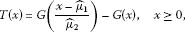(40)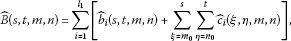(41)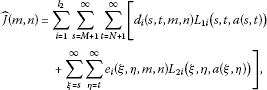(42)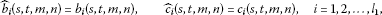(43)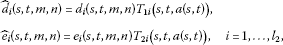(44)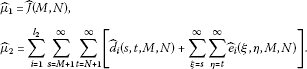(45)

Proof Denote

$\begin{array}{rcl}v\left(m,n\right)& =& \sum _{i=1}^{{l}_{1}}\sum _{s=m+1}^{\mathrm{\infty }}\sum _{t=n+1}^{\mathrm{\infty }}\left[{b}_{i}\left(s,t,m,n\right)\phi \left(u\left(s,t\right)\right)+\sum _{\xi =s}^{\mathrm{\infty }}\sum _{\eta =t}^{\mathrm{\infty }}{c}_{i}\left(\xi ,\eta ,m,n\right)\phi \left(u\left(\xi ,\eta \right)\right)\right]\\ +\sum _{i=1}^{{l}_{2}}\sum _{s=M+1}^{\mathrm{\infty }}\sum _{t=N+1}^{\mathrm{\infty }}\left[{d}_{i}\left(s,t,m,n\right){L}_{1i}\left(s,t,\varphi \left(u\left(s,t\right)\right)\right)\\ +\sum _{\xi =s}^{\mathrm{\infty }}\sum _{\eta =t}^{\mathrm{\infty }}{e}_{i}\left(\xi ,\eta ,m,n\right){L}_{2i}\left(\xi ,\eta ,\varphi \left(u\left(\xi ,\eta \right)\right)\right)\right].\end{array}$

Then we have

$u\left(m,n\right)\le {\varphi }^{-1}\left(a\left(m,n\right)+v\left(m,n\right)\right).$
(46)

So,

$\begin{array}{rcl}v\left(m,n\right)& \le & \sum _{i=1}^{{l}_{1}}\sum _{s=m+1}^{\mathrm{\infty }}\sum _{t=n+1}^{\mathrm{\infty }}\left\{{b}_{i}\left(s,t,m,n\right)\phi \left[{\varphi }^{-1}\left(a\left(s,t\right)+v\left(s,t\right)\right)\right]\\ +\sum _{\xi =s}^{\mathrm{\infty }}\sum _{\eta =t}^{\mathrm{\infty }}{c}_{i}\left(\xi ,\eta ,m,n\right)\phi \left[{\varphi }^{-1}\left(a\left(\xi ,\eta \right)+v\left(\xi ,\eta \right)\right)\right]\right\}\\ +\sum _{i=1}^{{l}_{2}}\sum _{s=M+1}^{\mathrm{\infty }}\sum _{t=N+1}^{\mathrm{\infty }}\left\{{d}_{i}\left(s,t,m,n\right){L}_{1i}\left(s,t,a\left(s,t\right)+v\left(s,t\right)\right)\\ +\sum _{\xi =s}^{\mathrm{\infty }}\sum _{\eta =t}^{\mathrm{\infty }}{e}_{i}\left(\xi ,\eta ,m,n\right){L}_{2i}\left(\xi ,\eta ,a\left(\xi ,\eta \right)+v\left(\xi ,\eta \right)\right)\right\}\\ =& \sum _{i=1}^{{l}_{1}}\sum _{s=m+1}^{\mathrm{\infty }}\sum _{t=n+1}^{\mathrm{\infty }}\left\{{b}_{i}\left(s,t,m,n\right)\phi \left[{\varphi }^{-1}\left(a\left(s,t\right)+v\left(s,t\right)\right)\right]\\ +\sum _{\xi =s}^{\mathrm{\infty }}\sum _{\eta =t}^{\mathrm{\infty }}{c}_{i}\left(\xi ,\eta ,m,n\right)\phi \left[{\varphi }^{-1}\left(a\left(\xi ,\eta \right)+v\left(\xi ,\eta \right)\right)\right]\right\}\\ +\sum _{i=1}^{{l}_{2}}\sum _{s=M+1}^{\mathrm{\infty }}\sum _{t=N+1}^{\mathrm{\infty }}\left\{{d}_{i}\left(s,t,m,n\right)\left[{L}_{1i}\left(s,t,a\left(s,t\right)+v\left(s,t\right)\right)\\ -{L}_{1i}\left(s,t,a\left(s,t\right)\right)+{L}_{1i}\left(s,t,a\left(s,t\right)\right)\right]\\ +\sum _{\xi =s}^{\mathrm{\infty }}\sum _{\eta =t}^{\mathrm{\infty }}{e}_{i}\left(\xi ,\eta ,m,n\right)\left[{L}_{2i}\left(\xi ,\eta ,a\left(\xi ,\eta \right)+v\left(\xi ,\eta \right)\right)\\ -{L}_{2i}\left(\xi ,\eta ,a\left(\xi ,\eta \right)\right)+{L}_{2i}\left(\xi ,\eta ,a\left(\xi ,\eta \right)\right)\right]\right\}\\ \le & \sum _{i=1}^{{l}_{1}}\sum _{s=m+1}^{\mathrm{\infty }}\sum _{t=n+1}^{\mathrm{\infty }}\left\{{b}_{i}\left(s,t,m,n\right)\phi \left[{\varphi }^{-1}\left(a\left(s,t\right)+v\left(s,t\right)\right)\right]\\ +\sum _{\xi =s}^{\mathrm{\infty }}\sum _{\eta =t}^{\mathrm{\infty }}{c}_{i}\left(\xi ,\eta ,m,n\right)\phi \left[{\varphi }^{-1}\left(a\left(\xi ,\eta \right)+v\left(\xi ,\eta \right)\right)\right]\right\}\\ +\sum _{i=1}^{{l}_{2}}\sum _{s=M+1}^{\mathrm{\infty }}\sum _{t=N+1}^{\mathrm{\infty }}\left\{{d}_{i}\left(s,t,m,n\right)\left[{T}_{1i}\left(s,t,a\left(s,t\right)\right)v\left(s,t\right)+{L}_{1i}\left(s,t,a\left(s,t\right)\right)\right]\\ +\sum _{\xi =s}^{\mathrm{\infty }}\sum _{\eta =t}^{\mathrm{\infty }}{e}_{i}\left(\xi ,\eta ,m,n\right)\left[{T}_{2i}\left(\xi ,\eta ,a\left(\xi ,\eta \right)\right)v\left(\xi ,\eta \right)+{L}_{2i}\left(\xi ,\eta ,a\left(\xi ,\eta \right)\right)\right]\right\}\\ =& H\left(m,n\right)+\sum _{i=1}^{{l}_{1}}\sum _{s=m+1}^{\mathrm{\infty }}\sum _{t=n+1}^{\mathrm{\infty }}\left\{{b}_{i}\left(s,t,m,n\right)\phi \left[{\varphi }^{-1}\left(a\left(s,t\right)+v\left(s,t\right)\right)\right]\\ +\sum _{\xi =s}^{\mathrm{\infty }}\sum _{\eta =t}^{\mathrm{\infty }}{c}_{i}\left(\xi ,\eta ,m,n\right)\phi \left[{\varphi }^{-1}\left(a\left(\xi ,\eta \right)+v\left(\xi ,\eta \right)\right)\right]\right\},\end{array}$
(47)

where $\stackrel{ˆ}{H}\left(m,n\right)=\stackrel{ˆ}{J}\left(m,n\right)+{\sum }_{i=1}^{{l}_{2}}{\sum }_{s=M+1}^{\mathrm{\infty }}{\sum }_{t=N+1}^{\mathrm{\infty }}\left\{{\stackrel{ˆ}{d}}_{i}\left(s,t,m,n\right)v\left(s,t\right)+{\sum }_{\xi =s}^{\mathrm{\infty }}{\sum }_{\eta =t}^{\mathrm{\infty }}{\stackrel{ˆ}{e}}_{i}\left(\xi ,\eta ,m,n\right)v\left(\xi ,\eta \right)\right\}$, and $\stackrel{ˆ}{J}\left(m,n\right)$ is defined in (42). Then similar to the process of (21)-(23), we obtain

$v\left(m,n\right)\le {G}^{-1}\left[G\left(\stackrel{ˆ}{H}\left(M,N\right)\right)+\sum _{s=m+1}^{\mathrm{\infty }}\sum _{t=n+1}^{\mathrm{\infty }}\stackrel{ˆ}{B}\left(s,t,m,n\right)\right],$
(48)

and

$H\left(M,N\right)\le {T}^{-1}\left[\sum _{s=M+1}^{\mathrm{\infty }}\sum _{t=N+1}^{\mathrm{\infty }}\stackrel{ˆ}{B}\left(s,t,M,N\right)\right].$
(49)

Combining (46), (48) and (49), we get the desired result. □

Theorem 2.7 Suppose $w\in {\mathrm{\wp }}_{+}\left(\mathrm{\Omega }\right)$, u, a, ${b}_{i}$, ${c}_{i}$, ${d}_{i}$, ${e}_{i}$, φ, ϕ are defined as in Theorem  2.3, and ${L}_{ji}$, ${T}_{ji}$, $j=1,2$, $i=1,2,\dots ,{l}_{2}$ are defined as in Theorem  2.6. If for $\left(m,n\right)\in \mathrm{\Omega }$, $u\left(m,n\right)$ satisfies

$\begin{array}{rcl}\varphi \left(u\left(m,n\right)\right)& \le & a\left(m,n\right)+\sum _{s=m+1}^{\mathrm{\infty }}w\left(s,n\right)\varphi \left(u\left(m,n\right)\right)\\ +\sum _{i=1}^{{l}_{1}}\sum _{s=m+1}^{\mathrm{\infty }}\sum _{t=n+1}^{\mathrm{\infty }}\left[{b}_{i}\left(s,t,m,n\right)\phi \left(u\left(s,t\right)\right)+\sum _{\xi =s}^{\mathrm{\infty }}\sum _{\eta =t}^{\mathrm{\infty }}{c}_{i}\left(\xi ,\eta ,m,n\right)\phi \left(u\left(\xi ,\eta \right)\right)\right]\\ +\sum _{i=1}^{{l}_{2}}\sum _{s=M+1}^{\mathrm{\infty }}\sum _{t=N+1}^{\mathrm{\infty }}\left[{d}_{i}\left(s,t,m,n\right){L}_{1i}\left(s,t,\varphi \left(u\left(s,t\right)\right)\right)\\ +\sum _{\xi =s}^{\mathrm{\infty }}\sum _{\eta =t}^{\mathrm{\infty }}{e}_{i}\left(\xi ,\eta ,m,n\right){L}_{2i}\left(\xi ,\eta ,\varphi \left(u\left(\xi ,\eta \right)\right)\right)\right],\end{array}$

then

$\begin{array}{rcl}u\left(m,n\right)& \le & {\varphi }^{-1}\left\{a\left(m,n\right)+{G}^{-1}\left\{G\left({T}^{-1}\left[\sum _{s=M+1}^{\mathrm{\infty }}\sum _{t=N+1}^{\mathrm{\infty }}\stackrel{˜}{B}\left(s,t,M,N\right)\right]\right)\\ +\sum _{s=m+1}^{\mathrm{\infty }}\sum _{t=n+1}^{\mathrm{\infty }}\stackrel{˜}{B}\left(s,t,m,n\right)\right\}\right\}\end{array}$

provided that T is increasing, where G is defined in (4), and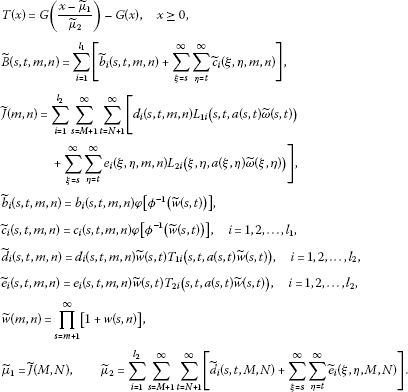The proof for Theorem 2.7 is similar to the combination of Theorem 2.5 and Theorem 2.6, and we omit the details here.

Remark 2.8 We note that the inequalities established in Theorems 2.3, 2.5-2.7 are essentially different from the results in  as the left-hand side of the inequalities established here is an arbitrary function $\varphi \left(u\right)$. Furthermore, if we set $\varphi \left(u\right)={u}^{p}$, $a\left(m,n\right)=0$, then Theorem 2.5 reduces to [, Theorem 2.5].

## 3 Applications

In this section, we present some applications for the results established above. Similar to the applications in , we research a certain Volterra-Fredholm sum-difference equation and derive some new bounds for its solutions.

Example Consider the following Volterra-Fredholm type infinite sum-difference equation:

$\begin{array}{rcl}{u}^{p}\left(m,n\right)& =& \sum _{s=m+1}^{\mathrm{\infty }}\sum _{t=n+1}^{\mathrm{\infty }}\left[{F}_{1}\left(s,t,m,n,u\left(s,t\right)\right)+\sum _{\xi =s}^{\mathrm{\infty }}\sum _{\eta =t}^{\mathrm{\infty }}{F}_{2}\left(\xi ,\eta ,m,n,u\left(\xi ,\eta \right)\right)\right]\\ +\sum _{s=M+1}^{\mathrm{\infty }}\sum _{t=N+1}^{\mathrm{\infty }}\left[{G}_{1}\left(s,t,m,n,u\left(s,t\right)\right)\\ +\sum _{\xi =s}^{\mathrm{\infty }}\sum _{\eta =t}^{\mathrm{\infty }}{G}_{2}\left(\xi ,\eta ,m,n,u\left(\xi ,\eta \right)\right)\right],\end{array}$
(50)

where $u\in \mathrm{\wp }\left(\mathrm{\Omega }\right)$, $p\ge 1$ is an odd number, ${F}_{i},{G}_{i}:{\mathrm{\Omega }}^{2}×\mathbb{R}\to \mathbb{R}$, $i=1,2$.

Theorem 3.1 Suppose $u\left(m,n\right)$ is a solution of (50), and $|{F}_{1}\left(s,t,m,n,u\right)|\le {f}_{1}\left(s,t,m,n\right){|u|}^{\frac{p}{2}}$, $|{F}_{2}\left(s,t,m,n,u\right)|\le {f}_{2}\left(s,t,m,n\right){|u|}^{\frac{p}{2}}$, $|{G}_{1}\left(s,t,m,n,u\right)|\le {g}_{1}\left(s,t,m,n\right){|u|}^{p}$, $|{G}_{2}\left(s,t,m,n,u\right)|\le {g}_{2}\left(s,t,m,n\right){|u|}^{p}$, ${f}_{i},{g}_{i}\in {\mathrm{\wp }}_{+}\left({\mathrm{\Omega }}^{2}\right)$, $i=1,2$, ${f}_{i}$, ${g}_{i}$ are nondecreasing in the last two variables, and there is at least one function among ${g}_{1}$, ${g}_{2}$ not equivalent to zero, then we have

$u\left(m,n\right)\le {4}^{-\frac{1}{p}}{\left\{\frac{\sqrt{\mu }}{1-\sqrt{\mu }}\sum _{s=M+1}^{\mathrm{\infty }}\sum _{t=N+1}^{\mathrm{\infty }}B\left(s,t,M,N\right)+\sum _{s=m+1}^{\mathrm{\infty }}\sum _{t=n+1}^{\mathrm{\infty }}B\left(s,t,m,n\right)\right\}}^{\frac{2}{p}}$
(51)

provided that $\mu <1$, where

$\begin{array}{c}B\left(s,t,m,n\right)={f}_{1}\left(s,t,m,n\right)+\sum _{\xi =s}^{\mathrm{\infty }}\sum _{\eta =t}^{\mathrm{\infty }}{f}_{2}\left(\xi ,\eta ,m,n\right),\hfill \\ \mu =\sum _{s=M+1}^{\mathrm{\infty }}\sum _{t=N+1}^{\mathrm{\infty }}\left[{g}_{1}\left(s,t,M,N\right)+\sum _{\xi =s}^{\mathrm{\infty }}\sum _{\eta =t}^{\mathrm{\infty }}{g}_{2}\left(\xi ,\eta ,M,N\right)\right].\hfill \end{array}$

Proof From (50) we have

$\begin{array}{rcl}{|u\left(m,n\right)|}^{p}& \le & \sum _{s=m+1}^{\mathrm{\infty }}\sum _{t=n+1}^{\mathrm{\infty }}\left[|{F}_{1}\left(s,t,m,n,u\left(s,t\right)\right)|+\sum _{\xi =s}^{\mathrm{\infty }}\sum _{\eta =t}^{\mathrm{\infty }}|{F}_{2}\left(\xi ,\eta ,m,n,u\left(\xi ,\eta \right)\right)|\right]\\ +\sum _{s={m}_{0}}^{M-1}\sum _{t={n}_{0}}^{M-1}\left[|{G}_{1}\left(s,t,m,n,u\left(s,t\right)\right)|+\sum _{\xi =s}^{\mathrm{\infty }}\sum _{\eta =t}^{\mathrm{\infty }}|{G}_{2}\left(\xi ,\eta ,m,n,u\left(\xi ,\eta \right)\right)|\right]\\ \le & |a\left(m,n\right)|+\sum _{s=m+1}^{\mathrm{\infty }}\sum _{t=n+1}^{\mathrm{\infty }}\left[{f}_{1}\left(s,t,m,n\right){|u\left(s,t\right)|}^{\frac{p}{2}}\\ +\sum _{\xi =s}^{\mathrm{\infty }}\sum _{\eta =t}^{\mathrm{\infty }}{f}_{2}\left(\xi ,\eta ,m,n\right){|u\left(\xi ,\eta \right)|}^{\frac{p}{2}}\right]\\ +\sum _{s=M+1}^{\mathrm{\infty }}\sum _{t=N+1}^{\mathrm{\infty }}\left[{g}_{1}\left(s,t,m,n\right){|u\left(s,t\right)|}^{p}\\ +\sum _{\xi =s}^{\mathrm{\infty }}\sum _{\eta =t}^{\mathrm{\infty }}{g}_{2}\left(\xi ,\eta ,m,n\right){|u\left(\xi ,\eta \right)|}^{p}\right].\end{array}$
(52)

Define $\varphi \left(u\right)={u}^{p}$, $\phi \left(u\right)={u}^{\frac{p}{2}}$, and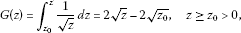(53)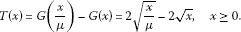(54)

Then by $\mu <1$, we have T is strictly increasing, and a suitable application of Theorem 2.3 (with $a\left(m,n\right)=0$ and ${l}_{1}={l}_{2}=1$) to (52) yields

$\begin{array}{rcl}u\left(m,n\right)& \le & {\varphi }^{-1}\left\{{G}^{-1}\left\{G\left({T}^{-1}\left[\sum _{s=M+1}^{\mathrm{\infty }}\sum _{t=N+1}^{\mathrm{\infty }}B\left(s,t,M,N\right)\right]\right)\\ +\sum _{s=m+1}^{\mathrm{\infty }}\sum _{t=n+1}^{\mathrm{\infty }}B\left(s,t,m,n\right)\right\}\right\}.\end{array}$
(55)

Combining (53)-(55), we can deduce the desired result. □

Theorem 3.2 Suppose $u\left(m,n\right)$ is a solution of (50), and $|{F}_{1}\left(s,t,m,n,u\right)|\le {f}_{1}\left(s,t,m,n\right){|u|}^{\frac{p}{3}}$, $|{F}_{2}\left(s,t,m,n,u\right)|\le {f}_{2}\left(s,t,m,n\right){|u|}^{\frac{p}{3}}$, $|{G}_{1}\left(s,t,m,n,u\right)|\le {g}_{1}\left(s,t,m,n\right){L}_{1}\left(s,t,{|u|}^{p}\right)$, $|{G}_{2}\left(s,t,m,n,u\right)|\le {g}_{2}\left(s,t,m,n\right){L}_{2}\left(s,t,{|u|}^{p}\right)$, where ${f}_{i}$, ${g}_{i}$, $i=1,2$ are defined as in Theorem  3.1, ${L}_{1},{L}_{2},{T}_{1},{T}_{2}:\mathrm{\Omega }×{\mathbb{R}}_{+}\to {\mathbb{R}}_{+}$ satisfies $0\le {L}_{i}\left(m,n,u\right)-{L}_{i}\left(m,n,v\right)\le {T}_{i}\left(m,n,v\right)\left(u-v\right)$ for $u\ge v\ge 0$ and ${L}_{i}\left(m,n,0\right)=0$, $i=1,2$, then we have

$u\left(m,n\right)\le {\left\{\frac{2}{3}\left[\frac{{\stackrel{ˆ}{\mu }}^{\frac{2}{3}}}{1-{\stackrel{ˆ}{\mu }}^{\frac{2}{3}}}\sum _{s=M+1}^{\mathrm{\infty }}\sum _{t=N+1}^{\mathrm{\infty }}\stackrel{ˆ}{B}\left(s,t,M,N\right)+\sum _{s=m+1}^{\mathrm{\infty }}\sum _{t=n+1}^{\mathrm{\infty }}\stackrel{ˆ}{B}\left(s,t,m,n\right)\right]\right\}}^{\frac{3}{2p}}$
(56)

provided that $\stackrel{ˆ}{\mu }<1$, where

$\begin{array}{c}\stackrel{ˆ}{B}\left(s,t,m,n\right)={f}_{1}\left(s,t,m,n\right)+\sum _{\xi =s}^{\mathrm{\infty }}\sum _{\eta =t}^{\mathrm{\infty }}{f}_{2}\left(\xi ,\eta ,m,n\right),\hfill \\ \stackrel{ˆ}{\mu }=\sum _{s=M+1}^{\mathrm{\infty }}\sum _{t=N+1}^{\mathrm{\infty }}\left[{g}_{1}\left(s,t,M,N\right){T}_{1}\left(s,t,0\right)+\sum _{\xi =s}^{\mathrm{\infty }}\sum _{\eta =t}^{\mathrm{\infty }}{g}_{2}\left(\xi ,\eta ,M,N\right){T}_{2}\left(\xi ,\eta ,0\right)\right].\hfill \end{array}$

Proof From (50) we have

$\begin{array}{rcl}{|u\left(m,n\right)|}^{p}& \le & \sum _{s=m+1}^{\mathrm{\infty }}\sum _{t=n+1}^{\mathrm{\infty }}\left[|{F}_{1}\left(s,t,m,n,u\left(s,t\right)\right)|+\sum _{\xi =s}^{\mathrm{\infty }}\sum _{\eta =t}^{\mathrm{\infty }}|{F}_{2}\left(\xi ,\eta ,m,n,u\left(\xi ,\eta \right)\right)|\right]\\ +\sum _{s=M+1}^{\mathrm{\infty }}\sum _{t=N+1}^{\mathrm{\infty }}\left[|{G}_{1}\left(s,t,m,n,u\left(s,t\right)\right)|+\sum _{\xi =s}^{\mathrm{\infty }}\sum _{\eta =t}^{\mathrm{\infty }}|{G}_{2}\left(\xi ,\eta ,m,n,u\left(\xi ,\eta \right)\right)|\right]\\ \le & \sum _{s=m+1}^{\mathrm{\infty }}\sum _{t=n+1}^{\mathrm{\infty }}\left[{f}_{1}\left(s,t,m,n\right){|u\left(s,t\right)|}^{\frac{p}{3}}+\sum _{\xi =s}^{\mathrm{\infty }}\sum _{\eta =t}^{\mathrm{\infty }}{f}_{2}\left(\xi ,\eta ,m,n\right){|u\left(\xi ,\eta \right)|}^{\frac{p}{3}}\right]\\ +\sum _{s=M+1}^{\mathrm{\infty }}\sum _{t=N+1}^{\mathrm{\infty }}\left[{g}_{1}\left(s,t,m,n\right){L}_{1}\left(s,t,{|u\left(s,t\right)|}^{p}\right)\\ +\sum _{\xi =s}^{\mathrm{\infty }}\sum _{\eta =t}^{\mathrm{\infty }}{g}_{2}\left(\xi ,\eta ,m,n\right){L}_{2}\left(\xi ,\eta ,{|u\left(\xi ,\eta \right)|}^{p}\right)\right]\\ \le & \sum _{s=m+1}^{\mathrm{\infty }}\sum _{t=n+1}^{\mathrm{\infty }}\left[{f}_{1}\left(s,t,m,n\right){|u\left(s,t\right)|}^{\frac{p}{3}}+\sum _{\xi =s}^{\mathrm{\infty }}\sum _{\eta =t}^{\mathrm{\infty }}{f}_{2}\left(\xi ,\eta ,m,n\right){|u\left(\xi ,\eta \right)|}^{\frac{p}{3}}\right]\\ +\sum _{s=M+1}^{\mathrm{\infty }}\sum _{t=N+1}^{\mathrm{\infty }}\left[{g}_{1}\left(s,t,m,n\right){T}_{1}\left(s,t,0\right){|u\left(s,t\right)|}^{p}\\ +\sum _{\xi =s}^{\mathrm{\infty }}\sum _{\eta =t}^{\mathrm{\infty }}{g}_{2}\left(\xi ,\eta ,m,n\right){T}_{2}\left(\xi ,\eta ,0\right){|u\left(\xi ,\eta \right)|}^{p}\right].\end{array}$
(57)

Define $\varphi \left(u\right)={u}^{p}$, $\phi \left(u\right)={u}^{\frac{p}{3}}$, and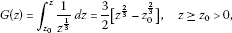(58)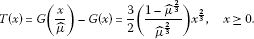(59)

Then by $\stackrel{ˆ}{\mu }<1$, we have T is strictly increasing, and a suitable application of Theorem 2.6 (with $\varphi \left(u\right)={u}^{p}$, $\phi \left(u\right)={u}^{\frac{p}{3}}$, $a\left(m,n\right)=0$ and ${l}_{1}={l}_{2}=1$) to (57) yields

$\begin{array}{rcl}u\left(m,n\right)& \le & {\varphi }^{-1}\left\{{G}^{-1}\left\{G\left({T}^{-1}\left[\sum _{s=M+1}^{\mathrm{\infty }}\sum _{t=N+1}^{\mathrm{\infty }}\stackrel{ˆ}{B}\left(s,t,M,N\right)\right]\right)\\ +\sum _{s=m+1}^{\mathrm{\infty }}\sum _{t=n+1}^{\mathrm{\infty }}\stackrel{ˆ}{B}\left(s,t,m,n\right)\right\}\right\}.\end{array}$
(60)

Combining (58)-(60), we can deduce the desired result. □

## References

1. Gronwall TH: Note on the derivatives with respect to a parameter of solutions of a system of differential equations. Ann. Math. 1919, 20: 292–296. 10.2307/1967124

2. Bellman R: The stability of solutions of linear differential equations. Duke Math. J. 1943, 10: 643–647. 10.1215/S0012-7094-43-01059-2

3. Ou-Iang L:The boundedness of solutions of linear differential equations ${y}^{″}+A\left(t\right){y}^{\prime }=0$. Shuxue Jinzhan 1957, 3: 409–418.

4. Pachpatte BG: Inequalities for Differential and Integral Equations. Academic Press, New York; 1998.

5. Cheung WS: Some new nonlinear inequalities and applications to boundary value problems. Nonlinear Anal. TMA 2006, 64: 2112–2128. 10.1016/j.na.2005.08.009

6. Zhao XQ, Zhao QX, Meng FW: On some new nonlinear discrete inequalities and their applications. J. Inequal. Pure Appl. Math. 2006., 7: Article ID 52

7. Yang EH: On some nonlinear integral and discrete inequalities related to Ou-Iang’s inequality. Acta Math. Sin. Engl. Ser. 1998, 14: 353–360. 10.1007/BF02580438

8. Cheung WS: Some discrete nonlinear inequalities and applications to boundary value problems for difference equations. J. Differ. Equ. Appl. 2004, 10: 213–223. 10.1080/10236190310001604238

9. Meng FW, Ji DH: On some new nonlinear discrete inequalities and their applications. J. Comput. Appl. Math. 2007, 208: 425–433. 10.1016/j.cam.2006.10.024

10. Ma QH, Pečarić J: Estimates on solutions of some new nonlinear retarded Volterra-Fredholm type integral inequalities. Nonlinear Anal. 2008, 69: 393–407. 10.1016/j.na.2007.05.027

11. Pachpatte BG: Inequalities applicable in the theory of finite differential equations. J. Math. Anal. Appl. 1998, 222: 438–459. 10.1006/jmaa.1998.5929

12. Pachpatte BG: On some new inequalities related to a certain inequality arising in the theory of differential equations. J. Math. Anal. Appl. 2000, 251: 736–751. 10.1006/jmaa.2000.7044

13. Cheung WS, Ma QH, Pečaric̀ J: Some discrete nonlinear inequalities and applications to difference equations. Acta Math. Sci., Ser. B 2008, 28: 417–430.

14. Deng SF: Nonlinear discrete inequalities with two variables and their applications. Appl. Math. Comput. 2010, 217: 2217–2225. 10.1016/j.amc.2010.07.022

15. Jiang FC, Meng FW: Explicit bounds on some new nonlinear integral inequality with delay. J. Comput. Appl. Math. 2007, 205: 479–486. 10.1016/j.cam.2006.05.038

16. Ma QH, Cheung WS: Some new nonlinear difference inequalities and their applications. J. Comput. Appl. Math. 2007, 202: 339–351. 10.1016/j.cam.2006.02.036

17. Ma QH: N -independent-variable discrete inequalities of Gronwall-Ou-Iang type. Ann. Differ. Equ. 2000, 16: 813–820.

18. Pang PYH, Agarwal RP: On an integral inequality and discrete analogue. J. Math. Anal. Appl. 1995, 194: 569–577. 10.1006/jmaa.1995.1318

19. Pachpatte BG: On some fundamental integral inequalities and their discrete analogues. J. Inequal. Pure Appl. Math. 2001., 2: Article ID 15

20. Meng FW, Li WN: On some new nonlinear discrete inequalities and their applications. J. Comput. Appl. Math. 2003, 158: 407–417. 10.1016/S0377-0427(03)00475-8

21. Ma QH: Some new nonlinear Volterra-Fredholm-type discrete inequalities and their applications. J. Comput. Appl. Math. 2008, 216: 451–466. 10.1016/j.cam.2007.05.021

22. Zheng B: Qualitative and quantitative analysis for solutions to a class of Volterra-Fredholm type difference equation. Adv. Differ. Equ. 2011., 2011: Article ID 30

23. Ma QH: Estimates on some power nonlinear Volterra-Fredholm type discrete inequalities and their applications. J. Comput. Appl. Math. 2010, 233: 2170–2180. 10.1016/j.cam.2009.10.002

24. Zheng B, Feng QH: Some new Volterra-Fredholm-type discrete inequalities and their applications in the theory of difference equations. Abstr. Appl. Anal. 2011., 2011: Article ID 584951

## Acknowledgements

The authors thank the referees very much for their careful comments and valuable suggestions on this paper.

## Author information

Authors

### Corresponding author

Correspondence to Bin Zheng.

### Competing interests

The authors declare that they have no competing interests.

### Authors’ contributions

BZ carried out the main part of this article. All authors read and approved the final manuscript.

## Rights and permissions

Reprints and Permissions

Zheng, B., Fu, B. Some Volterra-Fredholm type nonlinear discrete inequalities involving four iterated infinite sums. Adv Differ Equ 2012, 228 (2012). https://doi.org/10.1186/1687-1847-2012-228

• Accepted:

• Published:

• DOI: https://doi.org/10.1186/1687-1847-2012-228

### Keywords

• nonlinear discrete inequalities
• Volterra-Fredholm type inequalities
• sum-difference equations
• bounds